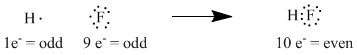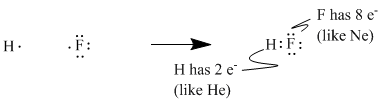# IM2. Lewis structures

In order to think about some of the compounds that can be made with this small collection of elements, we will need a way to visualize on paper how these atoms are connected with one another. At one time, people thought of the atoms in these compounds as charged spheres (such as oxygen anions, O2-, or carbon cations, C4+) that were simply pulled together by their opposing charges, like piles of sticky jellybeans. However, there are some limitations to this way of looking at compounds. We know that in most organic compounds, it is common to find two carbon atoms connected together, which is not likely to happen if all carbons are found as charged cations; in that case, they would simply repel each other.

In the early twentieth century, G.N. Lewis used some simple observations about the periodic table to propose an alternate model of how atoms can be bonded together. This model still influences the way we think about molecules today. First of all, Lewis noted that if you count up the number of electrons in all the atoms in almost any known molecular compound, such as water (H2O), ammonia (NH3) or carbon dioxide (CO2), you get an even number (10, 10 and 22, respectively, in these examples).Figure IM2.1. A tally of numbers of electrons in some small molecules.

From this observation he eventually proposed the notion that electrons are more stable if found in pairs. Two atoms with odd numbers of electrons may therefore gain stability if they come together to form an even number overall.

• molecules usually contain even numbers of total electrons

For example, hydrogen, with 1 electron, plus fluorine, with 9 electrons, come together to form hydrogen fluoride, with 10 electrons. The new pair of electrons that forms from the unpaired electron in fluorine and the single electron in hydrogen, Lewis thought, is the bond that holds the two atoms together.Figure IM2.2. A tally of numbers of electrons in a small molecule, hydrogen fluoride, showing the electrons as dots.

To simplify electron counting, one need only consider the valence electrons when thinking about bonding; one could ignore the core electrons, which are simply groups of electrons that already number the same as a noble gas. Thus, we consider fluorine's seven valence electrons instead of all nine; we consider nitrogen's five valence electrons and not all seven.Figure IM2.3. A tally of numbers of valence electrons in some small molecules.

• valence electrons are the electrons in an outer shell of an atom
• counting only valence electrons simplifies electron counting

Lewis made a couple of additions to this idea based on the layout of the periodic table. People were aware of the remarkable stability of the noble gases, and so it seemed that there was something particularly stable about the number of electrons found in these elements. These are even numbers, of course: 2 for helium, 10 for neon, 18 for argon, 36 for krypton and so on. In terms of valence electrons, neon has 8, argon has 8, krypton has 18 and so on. Lewis suggested that any atom could become more stable if it could share enough electrons from its neighbors to obtain the same electron count as a noble gas.Figure IM2.4. A tally of numbers of valence electrons in hydrogen fluoride.

• when an atom is found in a molecule, it usually has the same number of valence electrons as the noble gas in the same row
• this electron count includes unshared electrons as well as shared electrons
• for electron-counting, shared pairs are counted for both atoms that share the electrons

The fact that organic and biological chemistry, which constitutes a majority of familiar molecules, takes place mostly in the second row of the periodic table allows us to think of the Lewis model as "the octet rule," from the Latin word for eight: second-row elements form bonds so that they can have eight valence shell electrons, like neon.Figure IM2.5. A tally of numbers of electrons in some small molecules, using Lewis structures.

• most common biological or organic molecules are made from atoms in the second row of the periodic table
• it is common to see atoms with 8 electrons, like neon, in many molecules
• the Lewis "octet" rule says atoms frequently have 8 valence electrons in molecules
• atoms in other rows sometimes have larger numbers of electrons

Lewis structures are drawings that we use to show how the electrons in a molecule are arranged in a stable way. They let us verify that each atom in the molecule has an octet.

Note that a pair of electrons that forms a bond is usually written as a line instead of a pair of dots. This modification of a Lewis structure is sometimes called a Kekule structure. For the most part, Kekule structures will be used in these pages.

Exercise IM2.1

Try drawing Kekule structures for the following diatomic molecules:

a) Cl2 b) H2 c) HCl d) F2

What happens if a compound is actually an ion? A positive or negative charge tells you something about the structure. If there is a negative charge there must be an extra electron in the structure, so that the proton : electron ratio is no longer balanced. If the charge is two minus, there must be two extra electrons. For the same reason, a positive charge means an electron is missing from the structure.

• a negative charge means extra electron(s)
• a positive charge means missing electron(s)

Exercise IM2.2

Try drawing Kekule structures for the following ions:

a) HO- b) MgCl+ c) HS- d) OCl-